1. Terms

For this discussion we will use the following set of measurements: 45.66 45.66 45.68 45.65

Some terms we’ll encounter:

a. Discrepancy

The difference between any two measurements of the same quantity.

Example: the discrepancy between the highest and lowest measurements is 0.03

b. Most Probable Value (MPV)

This is a fancy way of saving “average” a.k.a. “mean”. In simplest form, it's the measurement sum divided by the number of measurements.Equation D-1 MPV: most probable vlaue meas: individual measurement n: number of measurements

Example: MPV = (45.66+45.66+45.68+45.65)/4 = 45.662

In some analyses where measurements of different accuracies are mixed you’ll see reference to the “weighted average.” This takes into account the measurement quality variations. For our purposes, we’ll stick with a straight (“unweighted”) average.

c. Residual (v)

The difference between a measurement and the MPV. This is like a discrepancy except it’s always compared against the MPV.

Example: Residual of the first measurement is 45.66-45.662 = -0.002

It doesn't really matter if the MPV is subtracted from the measurement or vice versa since we will ultimately square each so the mathematical sign goes away. Just be consistent: (measurement-MPV) or (MPV - measurement).

d. Normal Distribution Curve

If we compute all the residuals and graph their frequency we would get the traditional bell-shaped curve, Figure D-1. This curve is symmetric about the y-axis since some of the residuals will be positive and some negative. The graph is asymptotic- it approaches the x-axis but never touches it.Figure D-1 Normal Distribution Curve

Of course with only four measurements in this example, ours would be a pretty sorry looking bell. Just like flipping the coin, as you approach an infinite number of measurements your graph becomes a smooth curve.

e. Standard Deviation

On both sides of the curve is an inflection point: where the curve changes from convex to concave. These occur at ±σ along the x-axis, Figure D-2.Figure D-2 Standard Deviation

To compute the standard deviation:Equation D-2 σ: standard deviation v: residual n: number of measurements

You’ll also see another form of the equation:Equation D-3

What's the difference? This is a litle over-simplified, but basically:

Equation D-2 is used with a subset of all data.

Equation D-3 is used for an entire set of data.

Equation D-3 is sometimes referred to as the Standard Error because the data set is finite and can be used in its entirety to determine error present. For example: consider a factory making Do-dads. In the course of one day a finite number of Do-dads are produced as part of a batch. If we weigh each Do-dad, we would know the total weight error in the batch.

In measurement science, the only way we can quantify error present in an unknown quantity is to measure an infinite number of times. Since this is not practical, we instead settle for a limited number of measurements, a subset of infinity. So we use Equation D-2.

Note that as n increases, the difference between Equations D-2 and D-3 decreases. When n hits infinity, division by infinity or  (infinity-1) makes little difference.

Anyway, back to Standard Deviation, Equation D-2...

This area bounded by ±σ and the curve is ~68.3% of the total area under the entire normal distribution curve. What that means is ~68% of our measurements fall within ±σ of the MPV.

Standard deviation is an indicator of the measurement set precision: the smaller the standard deviation, the smaller the data spread, the better the precision. Figure D-3 demonstrates this for two different measurement sets.Figure D-3 Standard Deviation and Precision

f. Confidence Interval (CI)

A confidence interval is the degree of certainty that a value falls within a specific range. The standard deviation represents a 68.3% confidence interval: continuing measurements under similar conditions using similar equipment and procedures, we’re 68.3% confident that our results will fall within ±s of our MPV.

Other common CIs are 90% and 95%:

The 90% CI covers 90% of the area under the normal distribution curve. It’s roughly equal to 2 times the standard deviation so is often referred to as the 2 sigma (2σ) confidence. Measurements are expected to fall between ±2σ of the MPV 90% of the time.

The 95% CI, which includes 95% of the area under the curve, is roughly equal to 3 times the standard deviation so is sometimes referred to as the 3 sigma (3σ) confidence. Measurements are expected to fall between ±3σ of the MPV 95% of the time.

Hey, what about 100% confidence? After all, we spent all this money on expensive accurate equipment so we should be able to state 100% confidence, right? Well, you can. Since the normal distribution curve is asymptotic you can state with 100% confidence that your measurement will fall within ±infinity of the MPV.

g. Standard Error Of the Mean

This the expected error in the MPV. Since we don’t know the exact error present, this is only an indicator. It’s computed from:Equation D-4 EMPV: Error off the mean σ: standard deviation n: number of measurements

Note that it’s a function of the standard deviation and number of measurements. Theoretically, as the number of measurements increases, EMPV decreases: less error with more measurements since the random errors get more chances to cancel.

Standard error of the mean is an indicator of accuracy.

2. Examples

a. Measurement Set

Let's compute the statistics of our measurement set: 45.66 45.66 45.68 45.65

(Keep in mind that this is an absurdly small measurement set hardly worthy of statistical analysis. We're using it only to demonstrate the random error analysis process).

 Step (1) Step (2) Step (3) num value v = meas-MPV v2 1 45.66 45.66-45.662 = -0.002 0.000004 2 45.66 45.66-45.662 = -0.002 0.000004 3 45.68 45.68-45.662 = +0.018 0.000324 4 46.65 45.65-45.662 = -0.012 0.000144 sums 182.65 0.000476

Step (1) Compute MPV, Equation D-1

According to the rules of significant figures, the measurement sum has 5 sig fig.In addition to isolating errors, repeating measurements can also increase accuracy. In this case, we’ve gone from 0.01 units to 0.001 units. Of course, the more measurements made, the stronger that additional accuracy. Four measurements, as in our example, are not really enough for a true statistical picture.

Step (2) Compute the residualsSince this represents an intermediate computation, carry an additional digit. Residuals have the same units as the measurements.

Step (3) Square and sum the residuals

Keeping in mind the addition rule for significant figures, the measurement sum has 3 sig fig.

By the way, the term least squares comes from the fact the MPV is the number which results in the smallest (or least) sum of the squares of the residuals. Any other number will result in a larger sum.

Step (4) Compute the standard deviation, Equation D-2The standard deviation has the same units as the measurements.

Step (5) Compute the Error of the MPV, Equation D-4The error of the MPV has the same units as the measurements.

Step (6) Results

From Step (1), the MPV is good to 0.001

We should express the standard deviation and error of the mean to the same level of accuracy (resolution).

MPV = 45.662 ±0.013; EMPV = ±0.006

So what’s all this mean? Based on our measurements:

• The most probable value of the measured quantity is 45.662;
• 68% of our measurements fall within ±0.013 of the MPV;
• and the error in the MPV is expected to be ±0.006.

b. Angles

One of the problems working with angles is the tendency to carry insufficient digits when converting from deg-min-sec to decimal degrees. For some reason, three decimal places seems to be the norm but this can cause substantial rounding error computing measurement statistics.

To demonstrate this, lets look at a simple example consisting of 4 angles: 168°42'30", 168°42'10", 168°42'15",  and 168°42'25".

Case 1

Convert to and carry three decimal places.

 Angle Decimal Deg v; deg v2; deg2 168°42'30" 168.708 +0.002 0.000004 168°42'10" 168.703 -0.003 0.000009 168°42'15" 168.704 -0.002 0.000004 168°42'25" 168.707 +0.001 0.000001 sums: 674.822 0.000018The computations aren't too onerous (a byproduct of using only three decimal places). However, once the angles are converted to decimal degrees, it's difficult to interpret magnitudes of subsequent calculations. Residuals are in decimal degrees; what are those in terms of the original mixed units? It's hard to judge if the residuals (and MPV, SD, EMPV) make sense for the measurement set.

Case 2

Convert to and carry seven decimal places

 Angle Decimal Deg v; deg v2; deg2 168°42'30" 168.7083333 +0.0027777 0.0000077156 168°42'10" 168.7027778 -0.0027778 0.0000077162 168°42'15" 168.7041667 -0.0013889 0.0000019290 168°42'25" 168.7069444 +0.0013888 0.0000019288 sums: 674.8222222 0.0000192896While the process is the same as the first case, two things are readily apparent:

(1) There are a lot more numbers to write down and use in calculations, and,

(2) The additional four decimal places have a significant impact the MPV, SD, and EMPV values.

Conclusion? Carrying only three decimal places results in substantial intermediate rounding. As we learned in the Significant Figure chapter, carrying more digits than needed and rounding at the end means less biased results.

But seven decimal places are sooooo many to carry. Would six be enough? Five? Four? We discussed how to convert angles to decimal degrees to the correct number of sig fig in the Significant Figures chapter. Although we could determine how many decimal places are needed, we still have the same issue as before: it's difficult to mentally compare decimal degree values to deg-min-sec.

But there's a better, simpler way

Case 3

An angle is a mixed units quantity and usually, only the smallest unit changes, the others don't (more on that in a bit - be patient). Recognize a pattern: in our angles, each has 168° and 42'; only the seconds vary. We can simplify computations considerably if we work with just the seconds. To do that, subtract 168°42' (both of which are exact) from each angle.

 Angle Sec only v; sec v2; sec2 168°42'30" 30 +10 100 168°42'10" 10 -10 100 168°42'15" 15 -5 25 168°42'25" 25 +5 25 sums: 80 250Lookey there - the same exact results as Case 2! And the computations are soooo much easier (you could almost do them all in your heard). The residuals are in the smallest unit, seconds, so are easy to compare to their respective angle. We don't have to carry a lot of decimal places or figure out significant figures for the converted angles.

OK, but what about an angle set where the minutes vary? Let's use: 89°36'58", 89°36'55, 89°37'05', 89°37'10"

To work with only the seconds portion, subtract 89°36' from each angle. For the last two angles, the results are 01'05" and 01'10", respectively; write them as seconds: 65" and 70".

 Angle Sec only v; sec v2; sec2 89°36'58" 58 -4.0 +16.0 89°36'55" 55 -7.0 +49.0 89°37'05" 65 +3.0 +9.0 89°37'10" 70 +8.0 +64.0 sums: 248 138.0Could we have instead subtracted 89°37' from each angle? Sure:

 Angle Sec only v; sec v2; sec2 89°36'58" -02 -02"-02.0" = -04.0" +16.0 89°36'55" -05 -05"-02.0" = -07.0" +49.0 89°37'05" +05 +05"-02.0" = +03.0" +9.0 89°37'10" +10 +10"-02.0" = +08.0" +64.0 sums: +08 138.0The results are the same as before but this time we must keep track of negative numbers. It less error prone to subtract 89°36' instead of 89°37'.

3. The Danger of Including Mistakes

Let’s include a measurement with a mistake and see what happens.

Make the first measurement 46.66, an error of 1.00 units.

 num value v=meas=MPV v2 1 46.66 46.66-45.912 = +0.748 0.559504 2 45.66 45.66-45.912 = -0.252 0.063504 3 45.68 45.68-45.912 = -0.232 0.053824 4 46.65 45.65-45.912 = -0.262 0.068644 sums 183.65 0.745476Note how large the standard deviation and EMPV error become. That’s because both are affected by the huge increase in the residuals caused by pushing parts of the 1.00 unit error into all of them.

Always: get rid of mistakes before trying to analyze random errors.

4. What About Unresolved Systematic Errors?

Go back to our mistake-free measurement set: MPV = 45.662 ±0.013; EMPV = ±0.006.

What if these are distances measured with a steel tape and we find out later that the tape started at 1.00' instead of 0.00'? What happens to our analysis?

Well, the MPV changes because each measurement is 1.00' too large (e.g., 45.66 should be 44.66). You can recompute the MPV or just subtract 1.00' from it: MPV = 44.662

How about the standard deviation and MPV error?

They don’t change because the residuals don’t change: since each measurement and the MPV lose 1.00', the residuals stay the same.

But remember that with the systematic error present, the accuracy indivcator (EPMV) is still pretty low which implies good accuracy. Go back and review the targets, particularly Figure C-1(b). An unresolved systematic error will affect accuracy.

So accounting for the systematic error: MPV = 44.662 ±0.013; EMPV=±0.006. That’s the nice thing about systematic errors: you can often eliminate them by computation.

5. Comparing Different Measurement Sets

Two survey crews measure different horizontal angles multiple times; their results are shown in the table.

 Crew A Crew B 2 D/R 4 D/R Average Angle 128°18'15" 196°02'40" Std Dev ±00°00'12" ±00°00'14"

D/R means to measure an angle direct and reverse; each D/R set is 2 measurements

Which crew has better precision?

Crew A because their standard deviation is smaller.

Which crew has better accuracy?

We need to determine the expected error in each crew’s average angle. Use Equation D-4.

 Crew A:Crew B:Crew B has better accuracy since the expected error in their average angle is less.

6. Error Propagation

Usually we combine measurements together to compute other quantities. Errors in those measurements affect the accuracy of the resulting computation. This is what’s meant by error propagation. How the errors propagate into and affect the result depends on the type of calculations involved.

Three of the more common error propagations are Error of a Sum, Error of a Series, and Error of a Product.

a. Error of a Sum

This is the expected cumulative error when adding or subtracting measurements having individual errors.Equation D-5 ESum: Error of a sum Ei: Error of ith item

Example

A line is measured in three segments. The mean and error for each segment is shown in Figure D-4: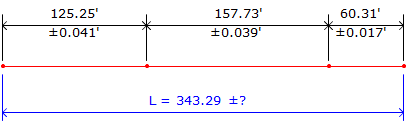Figure D-4 Error of a Sum

What is the error in the total length?

Substitute the individual errors into Equation D-5 and solve:Why not just add up all three errors and use that as the error for the entire line?

0.041'+0.039'+0.017' = ±0.097'

That would assume all three errors behave identically. Since each is ±, what's to say the total isn't (+0.041-0.039-0.017) or (-0.041+0.039-0.017) or... As a matter of fact, the range for the first error is ±0.041: it can be anything between -0.041 and +0.041, ditto for the others.

The beauty of random errors...

How about if we subtract numbers which have errors? Well, basically the same thing.Figure D-5 Error of a Sum

The error in the remaining length is:Why not subtract the square of the errors instead of adding them? Consider if both segment errors were the same, for example, ±0.50'; subtracting the square of the errors means the remainder would have no error. Remember that each individual error is ± so they don't behave in a straight algebraic fashion.

b. Error of a Series

This is used when there are multiple occurrences of the same expected error. This is typical when measurements with similar errors are multiplied or divided.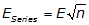Equation D-6

E is the consistent error

n is the number of times it occurs

Example

The interior angle sum of a five sided polygon, Figure D-6, is 540°00'00".Figure D-6 Error of a Series

A survey crew is able to measure angles consistently to an accuracy of ±0°00’10.” (nearest second). How close to 540°00'00" should they expect to be after measuring all five angles?

Substitute into and solve Equation D-6:We would expect the crew’s angle sum to be within 00°00'23" of 540°00'00".

c. Error of a product

This is the expected error in the product of multiplied or divided numbers.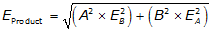Equation D-7

A, EA are the measurement of a quantity and its error

B, EB are the measurement of a second quantity and its error.

Notice that each product is the square of a quantity times the square of the error of the other quantity.

Example

The length and width of a parking lot are measured multiple times with the results shown in Figure D-7: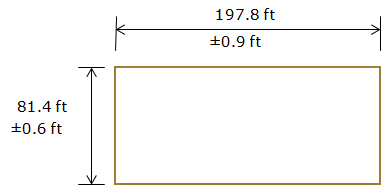Figure D-7 Error of a Product

The area of the parking lot isWhat is the error in that area? Substituting the dimensions and their errors in Equation D-7: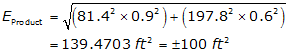d. Others

There are many different types of error propagation depending how measurements are combined. Sometimes a sensitivity analysis, discussed earlier, is an easier way to estimate a final error. We’ll discuss error effects more as we look at different measurement and computational processes.